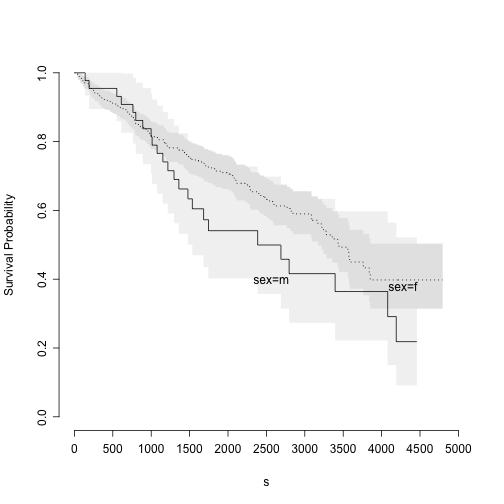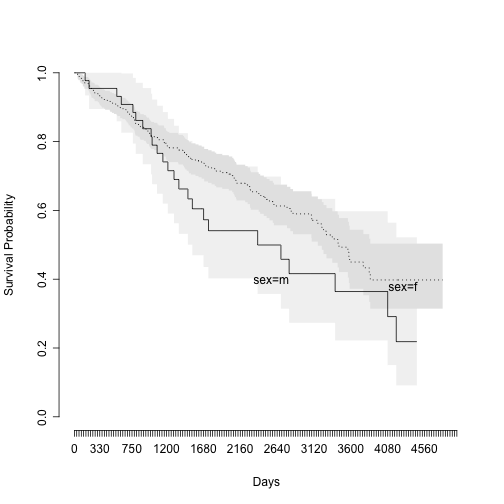# rms 4.2 survplot.survfit workaround

## Problem

rms::survplot() used to allow plotting a survfit object through survplot.survfit() function. However, this function has been deprecated as of version 4.2.

``````library(rms)
library(survival)
data(pbc)
``````

## Problem and workaround

``````## Create a survfit object.
objSurvfit <- survfit(formula = Surv(time,status == 2) ~ sex, data = pbc)

## This no longer works.
survplot(objSurvfit)
``````
``````## Error: no applicable method for 'survplot' applied to an object of class "survfit"
``````
``````
## Instead npsurv (nonparametric surv) function in rms is necessary.
objNpsurv <- npsurv(formula = Surv(time,status == 2) ~ sex, data = pbc)
class(objNpsurv)
``````
``````##  "npsurv"  "survfit"
``````
``````
## This can be plotted with survplot
survplot(objNpsurv)
````````````
## This really is a survfit object with additional data.
objNpsurv
``````
``````## Call: npsurv(formula = Surv(time, status == 2) ~ sex, data = pbc)
##
##       records n.max n.start events median 0.95LCL 0.95UCL
## sex=m      44    44      44     24   2386    1536      NA
## sex=f     374   374     374    137   3445    3170      NA
``````
``````
## So this hack kind of works, too.
class(objSurvfit) <- c(class(objSurvfit), "npsurv")
survplot(objSurvfit)
``````